﻿ 深部开采隔水关键层在不同高跨比下的力学解对比

深部开采隔水关键层在不同高跨比下的力学解对比Comparison of Mechanical Solutions of Water-Resistant Key Strata in Deep Mining under Different Height-Span Ratios

Abstract: In the practice of water inrush prevention and control in deep mining, the key water-repellent layer with different height-span ratio of coal floor and the water pulling force acting on the wa-ter-repellent layer are important factors to determine whether water inrush can be carried out. In this paper, the thickness and span ratio of the key layer of the water barrier are simulated as the height-to-span ratio of the beam. According to the mechanical characteristics of the fixed beam under different height-span ratios, two solutions of elastic mechanics and material mechanics are used to solve the key points of the fixed beam, to solve the stress of the two calculation results and FLAC3D simulation results, to explore whether the shallow beam theory is suitable for the stress analysis of the key layer, and apply it to the application of the actual water-resistant key layer. The results show that when the height span ratio is less than 0.2 for shallow beams, the normal stress error of elastic mechanics solution is about 11%~20%, and the normal stress error of material mechanics solution is 20%~30%. Comparatively, the elastic mechanics solution is more accurate, and the elastic mechanics solution of shallow beam theory is more suitable for key layers. When the height-span ratio is more than 0.2, the error of elastic mechanical solution appears “jump” phenomenon, and the error of material mechanical solution also increases gradually, and the wa-ter-proof key layer is no longer suitable for the shallow beam theory of both. The maximum error of shear stress solution is less than 11.5%, which is in line with engineering accuracy. To sum up, it is recommended to adopt the shallow beam theory of elastic mechanics solution for stress analysis of fixed beams in key layers under the condition that the height-span ratio is less than 0.2. The research results have important theoretical guiding significance for deep high-supported water mining safety and mine water inrush monitoring and imminent prediction.

1. 引言

2. 模型建立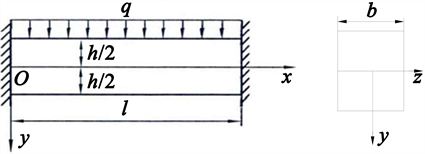Figure 1. Plane model of fixed beam at both ends

$y=-h/2:{\sigma }_{y}=0,{\tau }_{xy}=0$

$y=h/2:{\sigma }_{y}=-q,{\tau }_{xy}=0$

3. 两种算法解析解

3.1. 弹性力学解

$\begin{array}{l}{\sigma }_{x}=-\frac{1}{I}\left(\frac{1}{2}q{x}^{2}+{F}_{0}x\right)y+\frac{q}{3I}{y}^{3}+6{A}_{4}y+2{A}_{5}\\ {\sigma }_{y}=-\frac{q}{2I}\left(\frac{1}{3}{y}^{3}-\frac{1}{4}{h}^{2}y+\frac{{h}^{3}}{12}\right)\\ {\tau }_{xy}=-\frac{1}{2I}\left(qx+{F}_{0}\right)\left(\frac{{h}^{2}}{4}-{y}^{2}\right)\end{array}\right\}$

3.2. 材料力学解

$\begin{array}{l}{\sigma }_{x}=\frac{M\left(X\right)}{I}y\\ {\sigma }_{y}=-\frac{q}{2b}\left[1-3\frac{y}{h}+4{\left(\frac{y}{h}\right)}^{3}\right]\\ {\tau }_{xy}=\frac{3Q\left(X\right)}{2bh}×\left(1-\frac{4{y}^{2}}{{h}^{2}}\right)\end{array}\right\}$

4. 算例分析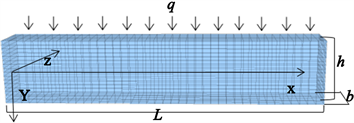Figure 2. Stress model of simulated beam in water-proof key layer

1) 当h/l = 0.1时，取h = 4, L = 40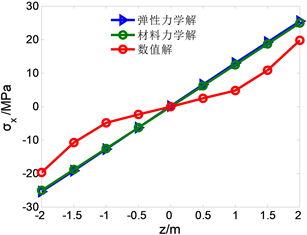Figure 3. Change with z when x = 0 m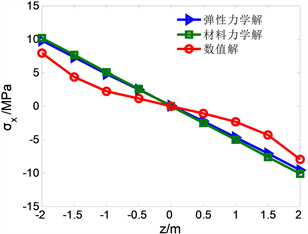Figure 4. Change with z when x = 15 m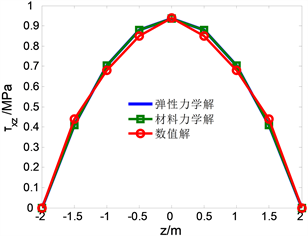Figure 5. Change with z when x = 15 m

2) 当h/l = 0.2时，取h = 8, L = 40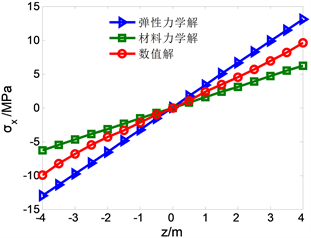Figure 6. Change with z when x = 0 m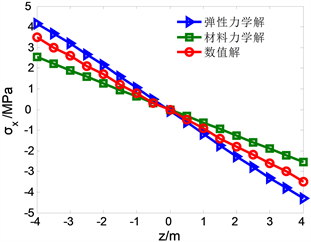Figure 7. Change with z when x = 15 m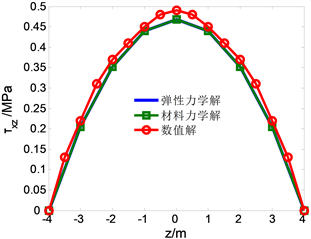Figure 8. Change with z when x = 15 m

3) 当h/l = 0.3时，取h = 12, L = 40Figure 9. Change with z when x = 0 m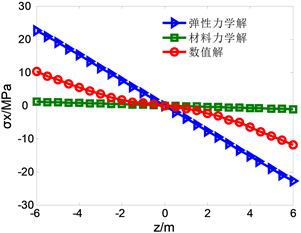Figure 10. Change with z when x = 15 mFigure 11. Change with z when x = 15 m

4) 当h/l = 0.4时，取h = 16, L = 40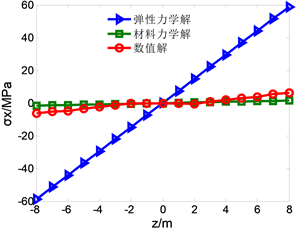Figure 12. Change with z when x = 0 m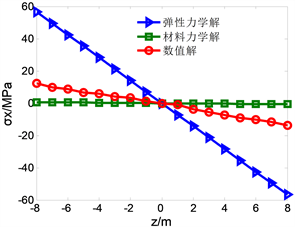Figure 13. Change with z when x = 15 m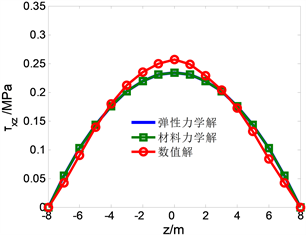Figure 14. Change with z when x = 15 mTable 1. Comparative analysis of tensile stress of two mechanical solutions under different height-span ratiosTable 2. Comparative analysis of elastic and material mechanical shear stress solutions under different height-span ratios

5. 结论

1) 数值计算表明，当h/l ≤ 0.2时，弹性力学解和数值解相差不大，在实际误差范围内。虽然在理论分析中材料力学对于杆件受力分析的方法在工程误差范围内，但弹性力学解略微精确，建议首选采用弹性力学解对隔水关键层进行受力分析．超过0.2时，两者浅梁理论不在适用隔水关键层。

2) 当将水压力近似看场均布荷载施加在关键层的时候，可以近似看出两端固定力学模型；由于均布荷载下固定梁的特点，下部受拉应力影响，而岩石抗拉强度一般较小，底板容易因开采扰动引起的应力重分布导致突水发生，故关键层的端部最底部的位置是防治底板突水的关键部位。而在x = 15 m处，靠近中层位置，弹性力学解浅梁解误差在11.3%相比材料力学解13.1%更为精确。在端点X = 0处两者误差相差不多，为0.4%。

3) 综合考虑 ${\sigma }_{x}$${\tau }_{xy}$ 随着xz的变化以及两种算法的精确程度，当高跨比不超过0.2时，弹性力学解应对浅梁分析更为贴切工程实际，且计算较为精确，在实际工程运用中，对于关键层的位移以及受力的内部应力变化有较好的理论支撑，可为底板突水的预测预报情况起到较好的指导作用。

 徐智敏. 深部开采底板破坏及高承拉突水模式、前兆与防治[D]: [博士学位论文]. 徐州: 中国矿业大学, 2010.

 杨天鸿, 唐春安, 谭志宏, 朱万成, 冯启言. 岩体破坏突水模型研究现状及突水预测预报研究发展趋势[J]. 岩石力学与工程学报, 2007, 26(2): 268-277.

 武强, 崔芳鹏, 赵苏启, 刘守强, 曾一凡, 谷亚威. 矿井水害类型划分及主要特征分析[J]. 煤炭学报, 2013, 38(4): 561-565.

 钱鸣高, 缪协兴, 许家林. 岩层控制中的关键层理论研究[J]. 煤炭学报, 1996(3): 2-7.

 刘广全, 孙科. 钢筋混凝土深梁研究综述[J]. 科技信息(科学教研), 2008(3): 88+107.

 Strutt, J.W. (1877) Theory of Sound. Macmillan Publications Co., Inc., Lon-don.

 Timoshenko, S.P. (1921) On the Correction for Shear of the Differential Equation for Transverse Vibration of Prismatic Bars. The London, Edinburgh, and Dublin Philosophical Magazine and Journal of Science, 41, 744-746.
https://doi.org/10.1080/14786442108636264

 Timoshenko, S.P. (1922) On the Transverse Vibration of Bars of Uniform Cross-Section. The London, Edinburgh, and Dublin Philosophical Magazine and Journal of Science, 43, 125-131
https://doi.org/10.1080/14786442208633855

 Han, S.M., Benaroya, H. and Wei, T. (1999) Dynam-ics of Transversely Vibrating Beams Using Four Engineering Theories. Journal of Sound and Vibration, 225, 935-988.
https://doi.org/10.1006/jsvi.1999.2257

 曲昭霞, 赵永刚, 秦慧峰. 非线性本构关系下两端固定梁的弯曲变形[J]. 甘肃科学学报, 2020, 32(1): 1-4.

 Lo, C.C. and Guffa, S.D. (1978) Bending of a Nonlinear Rectangular Beam in Large Deflection. Journal of Applied Mechanics, 45, 213-225.
https://doi.org/10.1115/1.3424238

 陈英昕, 吴思远, 李斯, 王正中, 吴守军. 考虑翘曲及挤压影响的深孔闸门主梁正应力解析解[J]. 水利水运工程学报, 2019(4): 116-123.

 张鹄志, 马哲霖, 黄海林, 金浩, 彭玮. 不同位移边界条件下钢筋混凝土深梁拓扑优化[J]. 工程设计学报, 2019, 26(6): 691-699.

 夏桂云, 曾庆元. 深梁理论的研究现状与工程应用[C]//中国力学学会力学史与方法论专业委员会. 第七届全国力学史与方法论学术研讨会力学与实践专刊. 中国力学学会力学史与方法论专业委员会: 中国力学学会, 2015: 18-32.

 詹春晓. 含固支端梁的理论分析[D]: [博士学位论文]. 合肥: 合肥工业大学, 2017.

 吴思远, 王正中, 曹炜林, 张雪才. 深孔钢闸门组合截面主梁应力计算方法[J]. 北京工业大学学报, 2018, 44(1): 97-103.

 徐芝纶. 弹性力学简明教程[M]. 北京: 高等教育出版社, 2002.

Top